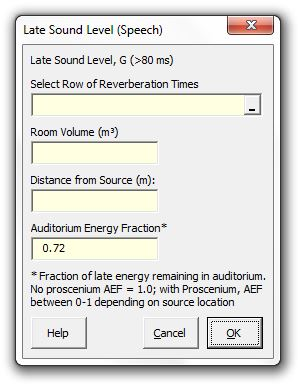### Strutt Help

Late Sound Level (Speech)    1/1, 1/3

Strutt|Auditorium Acoustics|Late Sound Level (Speech) calculates the late sound level in a drama auditorium using Barron's modified revised theoryThe modified version of revised theory allows for the case of a proscenium theatre in which a proportion of both the early and late sound energy will escape into the flytower.

The calculation uses an 'auditorium energy fraction' f which describes the proportion of the late energy that is retained in the auditorium.
(Energy entering the flytower is assumed to be effectively absorbed and is no longer considered in the calculation).

Barron provides guidance as to appropriate values for the auditorium fraction:

f=0.5 for the case of a source on the proscenium line facing across stage.
f=0.72 for the case of a source on the proscenium line facing into the auditorium.
f=1.0 for a theatre without a proscenium

Alternate values of f may be calculated based on the solid angle subtended by the proscenium for a particular source location, taking into account the source directivity and orientation. At present, this is beyond the capabilities of Strutt!

Note that the resulting level is expressed as strength (G) and can be converted to 'relative loudness' (i.e. relative to the source L_W) by subtracting 31 dB.

The late sound is calculated using the following relationship:

l = (31200 f T)/V e^-((0.04 r)/T) e^-(0.69/T), where

r is the distance from the source.
f is the auditorium energy fraction.
V is the auditorium volume.
T is the reverberation time.

Strutt calculates the total late sound level as:

G_(50ms-oo)=10log(l)

Reference: Barron Auditorium Acoustics and Architectural Design, 2nd edition, Appendix D.3